Showing 121 to 130 of 338 results.
0%## US states

• States
• United States
• US States
• Map
Practice the 50 states in the United States. Learn where the states are on the map and how they are spelled in English.
0%## Colors in German

• Colors
• Pronunciation
Practice the names of colors in German in multiple game types.
0%## Months in German

• Calendar
• Months
• Names
Learn what the months are called in German with several different types of questions in this game.
0%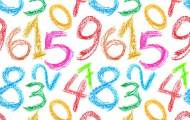## Numbers in German

• Numbers
• Spelling
• Digits
• Counting
Practice reading and spelling of the numbers from 0 to 20 in German.
0%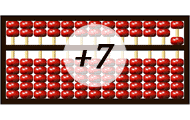• Logical thinking
• Abacus
Learn to count addition on an abacus. In order to be able to perform addition on an abacus you need to master the abacus basics. In this game you practice to carry extra digits when adding numbers.
0%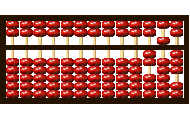## Abacus - Basics

• Logical thinking
• Mental calculation
• Abacus
Learn to count on an abacus online. In order to be able to count on an abacus you have to master the basics. In this game you train the values of the beads, the importance of the beam and the rods.
0%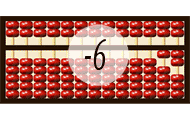## Abacus - Subtraction

• Logical thinking
• Subtraction
• Abacus
Practice subtraction on the abacus. In this game you practice to borrow when performing math calculation with subtraction. Please play the other abacus games before playing this game for better unders
0%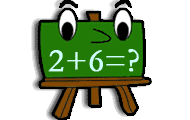• Numbers
• Mental calculation
Practice addition in math game with several levels of increasing difficulty. Add two numbers and find the correct sum.
0%• Fluency
This game will help students build fluency when adding within 10.
0%## Balance the Equations

• Equalities
• Balancing equations
• Subtraction
Use your addition and subtraction skills to balance the equations and win the game!
Weekly top list
1.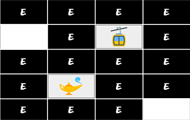Brain training
2.Brain training
3.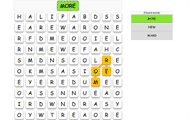English
4.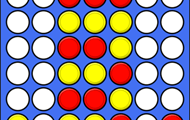Brain training
5.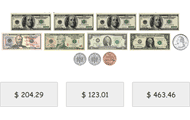Math